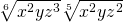# 9.8 Radicals of Mixed Index

Knowing that a radical has the same properties as exponents allows conversion of radicals to exponential form and then reduce according to the various rules of exponents is possible. This is shown in the following examples.

Example 9.8.1

Simplify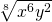.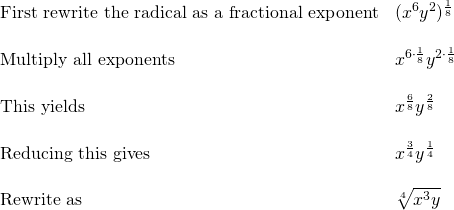Note: In Example 9.8.1, all exponents are reduced by the common factor 2. If there is a common factor in all exponents, reduce by dividing that common factor without having to convert to a different form.

Example 9.8.2

Simplify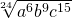.

For this radical, notice that each exponent has the common factor 3.

The solution is to divide each exponent by 3, which yields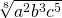.

When encountering problems where the index of the radicals do not match,convert each radical to individual exponents and use the properties of exponents to combine and then reduce the radicals.

Example 9.8.3

Simplify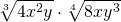.

First, convert each radical to a complete exponential form.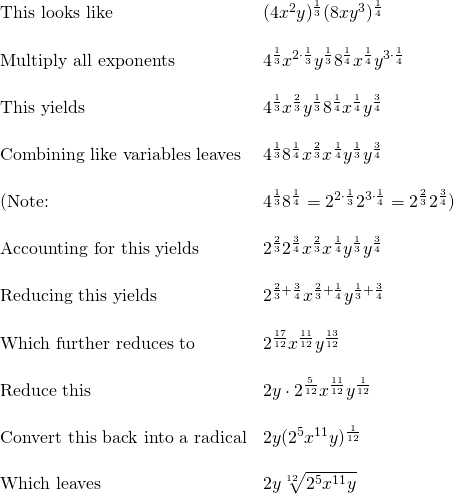The strategy of converting all radicals to exponents works for increasingly complex radicals.

Example 9.8.4

Simplify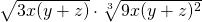.

First, convert each radical to a complete exponential form.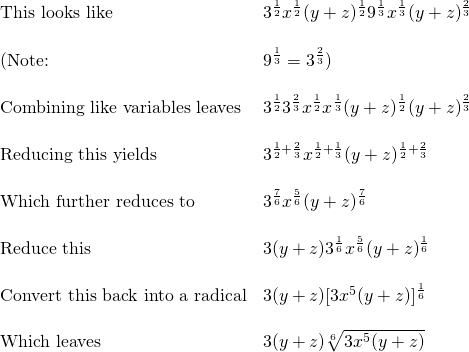# Questions

Reduce the following radicals. Leave as fractional exponents.

1.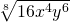2.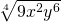3.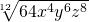4.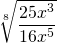5.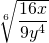6.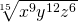7.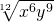8.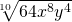9.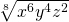10.11.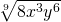12.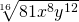Combine the following radicals. Leave as fractional exponents.

1.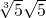2.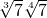3.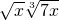4.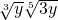5.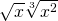6.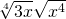7.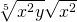8.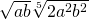9.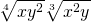10.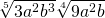11.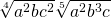12.